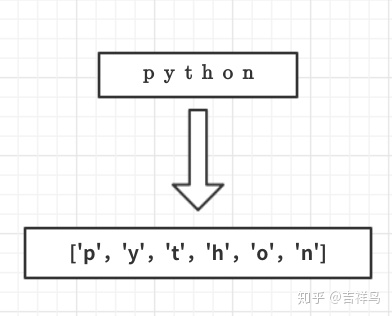# Python algorithm for “anagram” judgment problem

[TOC]

## problem description

“anagram” refers to a rearrangement of letters between two words

e.g. Heart and earth, Python and TYPHON

for simplicity, assume that the two words participating in the judgment are composed of only lowercase letters and have the same length

## problem goal

writes a bool function that takes two words as arguments to return whether they are anagram

## solution 1: check

verbatim

### solution idea

check the existence of the characters in word 1 one by one into word 2. Check the existence of the “check” mark (to prevent repetition)

if every character can be found, then these two words are an anagram, as long as a character is not found, it is not an anagram

### program tips

implements the “tick” mark: set the character corresponding to word 2 to None

since the string is immutable, you need to copy

into the list first## solution 2: sorting comparison

### problem solving idea

1. the two strings are sorted
2. and compared to see if they are equal

### program tips

1. turn STR list
2. sort
3. turn list STR
4. compare
```        ```def Method2(s1,s2):
# 字符串是不可变的无法排序，str转list
list1=list(s1)
list2=list(s2)
# 进行排序
list1.sort()
list2.sort()
# list 转 str
a = ''.join(list1)
b = ''.join(list2)
# 进行比较
if a==b:
return True
else:
return False

if __name__ == "__main__":
zzz2 = Method2('ablc','lcab')
print(zzz2)```
```

## solution 3: violent method

### problem solving idea

is just going to exhaust all the possible combinations

then sort all the characters that appear in s1, and see if s2 appears in the full sorted list

, there is no code attached here, violence is probably not a good algorithm here

## solution 4: count comparison

### problem solving idea

compares the number of occurrences of each character in the two words. If all 26 letters have the same number of occurrences, then the two words are an anagram

### program tips

```        ```def Method4(s1,s2):
c1 = * 26
c2 = * 26
for i in range(len(s1)):
pos = ord(s1[i])-ord('a')
c1[pos] = c1[pos]+1
for i in range(len(s2)):
pos = ord(s2[i])-ord('a')
c2[pos] = c2[pos]+1
stillok =True
j=0
while  stillok and j<26:
if c1[j]!=c2[j]:
stillok=False
j+=1
return stillok
if __name__ == "__main__":
zzz4 = Method4('ablc','lcab')
print(zzz4)```
```

, which is the best of the four solutions, T(n)=2n+26

the solution relies on two lists of 26 counters to hold the character count, so it requires more space than the previous three algorithms. In this case, space is exchanged for time